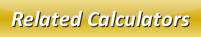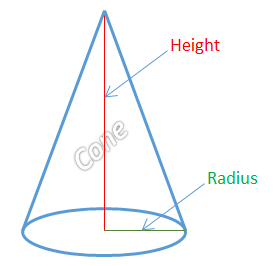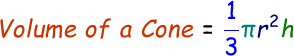# Volume of a Cone Calculator

To calculate volume of a cone we need to know the cone's height from vertex to the base and the base radius.Once we know both height and base radius, we can apply this below formula to find the volume of the given cone.where,
r = Base radius of the cone
h = Height of cone from vertex to base

In the below online volume of a cone calculator, enter the base radius and height of the cone and then click calculate to find the answer.

 Base Radius of the Cone: Height of Cone from Vertex to Base: Volume of Cone:

Latest Calculator Release

Average Acceleration Calculator

Average acceleration is the object's change in speed for a specific given time period. ...

Free Fall Calculator

When an object falls into the ground due to planet's own gravitational force is known a...

Torque Calculator

Torque is nothing but a rotational force. In other words, the amount of force applied t...

Average Force Calculator

Average force can be explained as the amount of force exerted by the body moving at giv...

Angular Displacement Calculator

Angular displacement is the angle at which an object moves on a circular path. It is de...